# Parallel and Perpendicular Lines

//Parallel and Perpendicular Lines

# Types of Lines

While parallel and perpendicular lines will be the primary focus of this chapter, there are other types of lines. Use the Types of Lines Foldable to distinguish between them.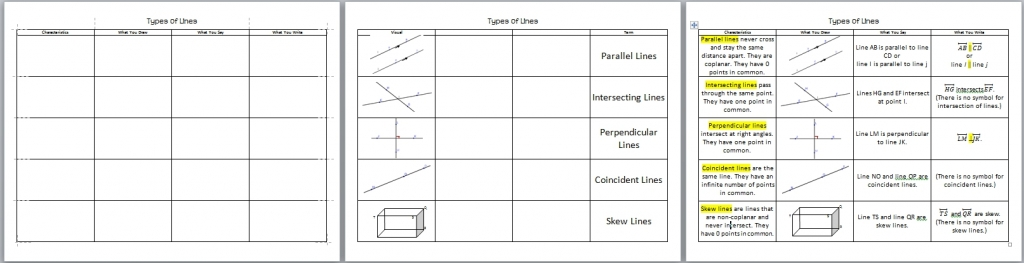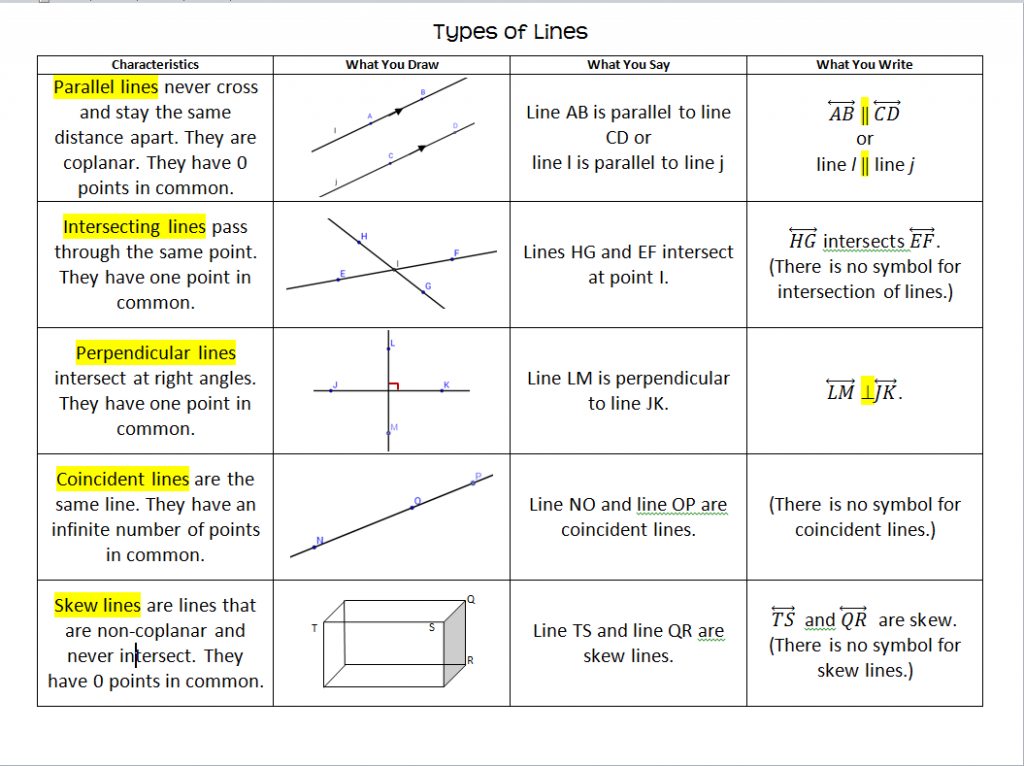# Types of Angles

When two lines are cut by a transversal, there are special names for the angles formed by the transversal and the lines. Use the Angles Formed by Transversal Foldable to master the vocabulary.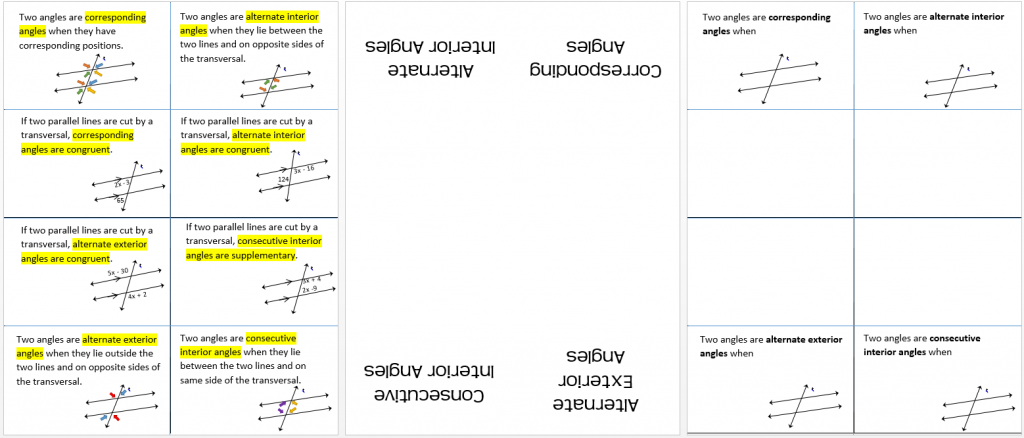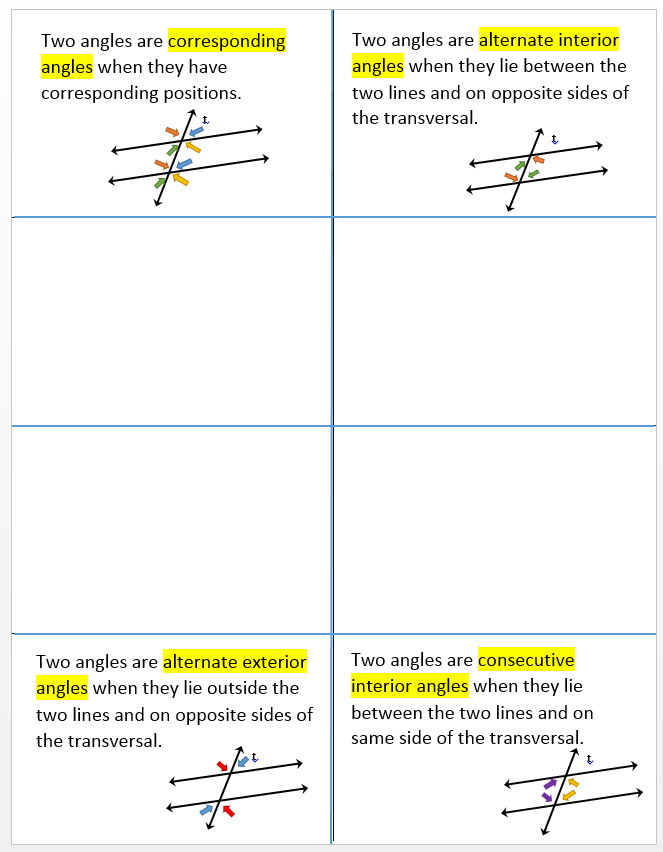Pairs of Lines and Angles Section 3.1 notes

# Parallel Lines and Transversals

## Exploring Parallel Lines and a Transversal

Have students use Geogebra to construct two parallel lines and a traversal. They then identify and measure corresponding, alternate interior, alternate exterior, and consecutive interior angles and make conjectures.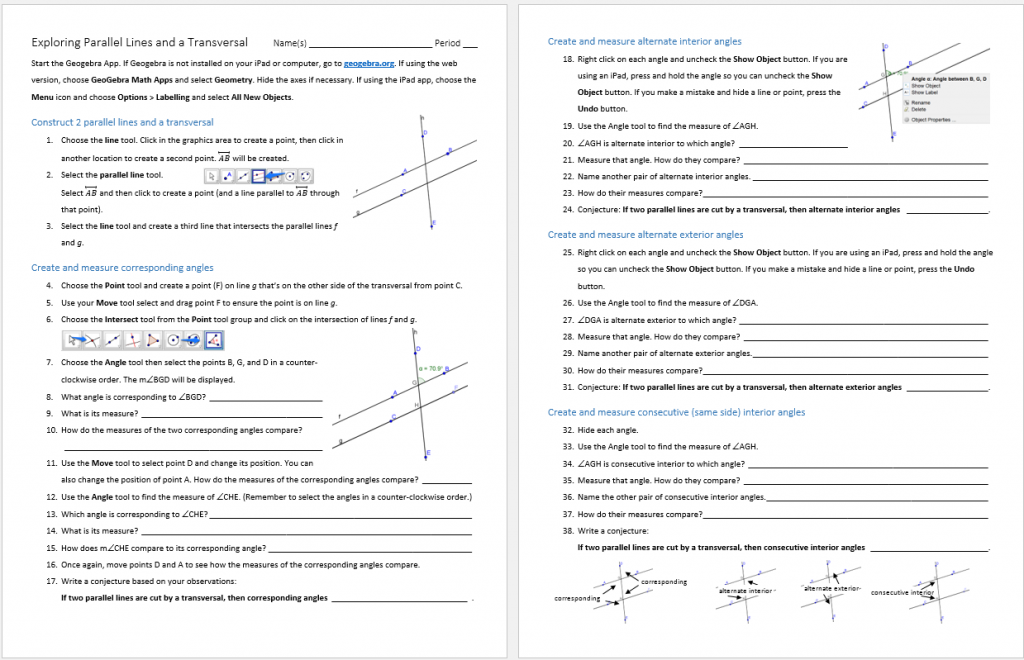### Alternate Exploration

Use the Geogebra activity below to explore the relationships between the special angles above when the lines that are cut by the transversal are parallel.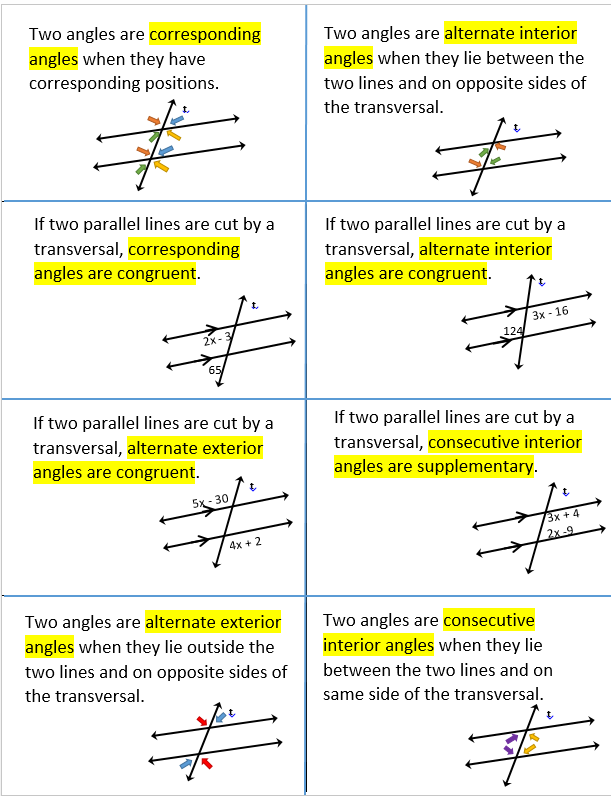Parallel Lines and Transversals Section 3.2notes

### Proving Lines are Parallel

Proofs with Parallel Lines Section 3.3notes

## Proofs with Perpendicular Lines

The Geogebra activity below helps underscore the fact that the distance from a point to a line is the length of the perpendicular segment from the point to the line.

Lesson 3-4 notes and worksheet

In class examples Proofs with Perpendicular Lines Lesson 3-4notes

## Equations of Parallel and Perpendicular Lines

Exploring Parallel and Perpendicular Lines Geogebra Activity will be used to learn how the slopes of parallel and perpendicular lines are related.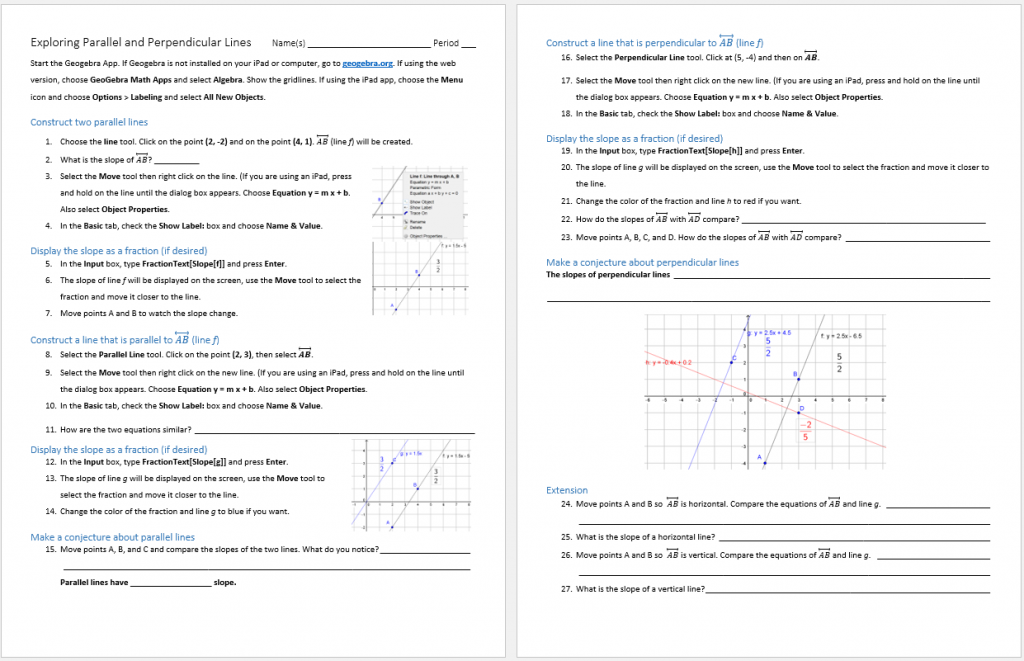Exploring Parallel and Perpendicular Lines Pencil Paper Activity will be used to learn how the slopes of parallel and perpendicular lines are related for those who don’t want to use technology.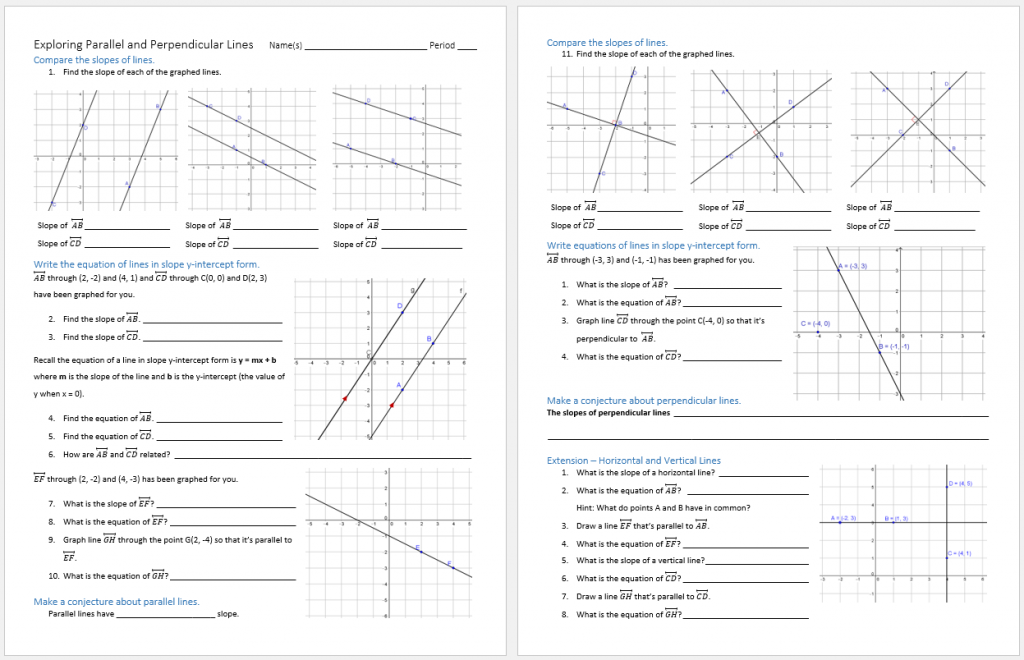The All About Slope Foldable can help you calculate slope given two points, find an equation in slope y-intercept form, use point slope form to find equations, and includes the relationships between parallel and perpendicular lines and their slopes.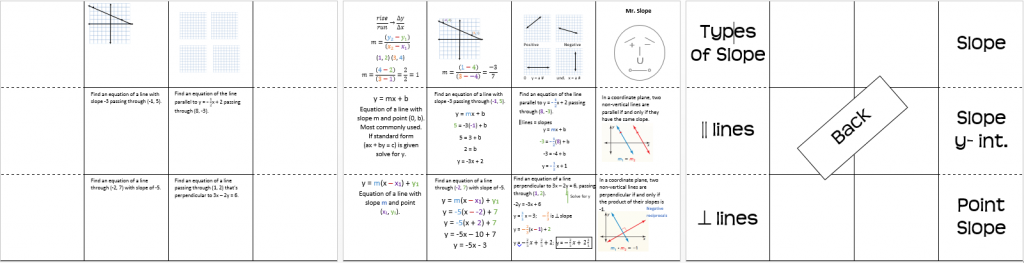When the inside is complete it will look similar to this.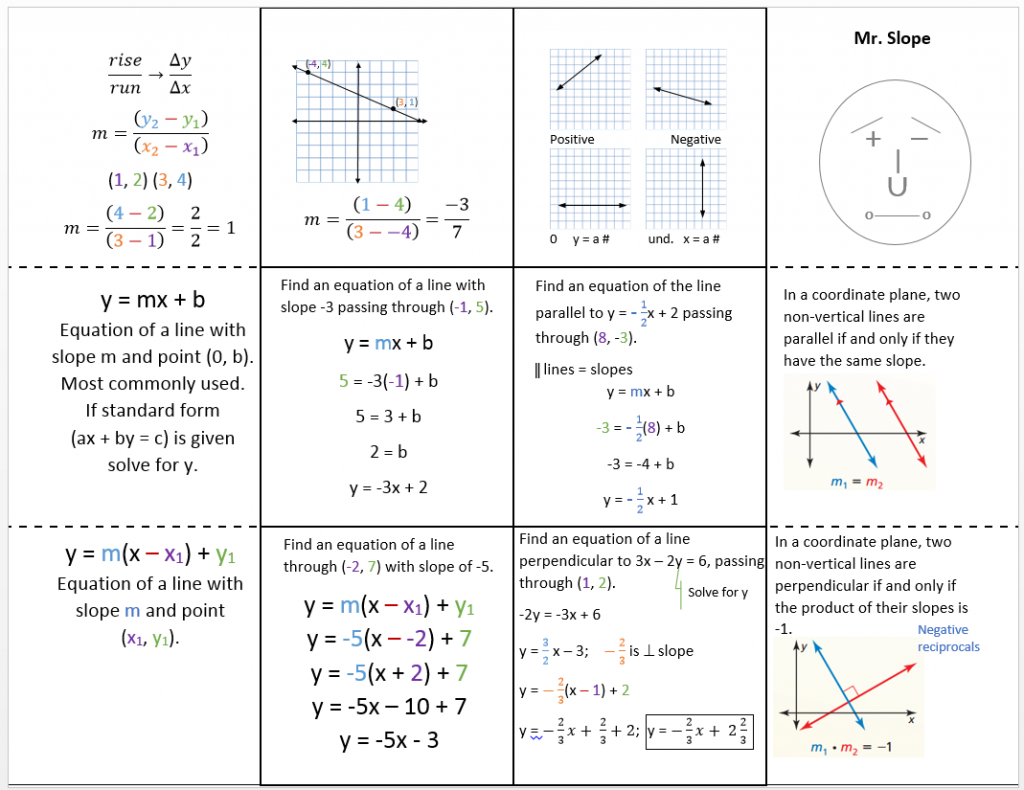A shout out to Mr. Fjelstrom for his Mr. Slope inspiration.

Equations of Parallel and Perpendicular Lines Lesson 3-5 notes day 2

Equations of Lines (3-5) ws

Equations of Parallel and Perpendicular Lines Lesson 3-5 notes day 3

By | 2021-11-01T18:41:27+00:00 October 5th, 2016|Geometry|2 Comments

### About the Author: Stacie Bender1.•